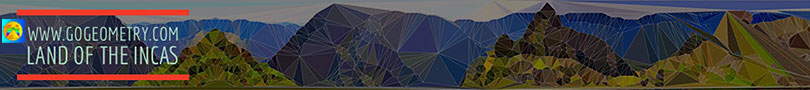Problem 120. Area of Triangles, Incenter, Excircles. Level: High School, SAT Prep, College
 In the figure below, given a triangle ABC, construct the incenter I and the excircles. Let be D, E, F, G, H, and J the tangent points of triangle ABC with its excircles. K, M, N, P, Q, and R are the intersection points of triangle ABC and ID, IE, IF, IG, IH, and IJ respectively. If S1, S2, S3, S4, S5, S6, S7, S8, and S9, are the areas of the shaded triangles, prove that S1+S2+S3+S4+S5+S6 = S7+S8+S9. View or post a solution..
 Home | Search | Problems | 111-120 | Email | By Antonio Gutierrez